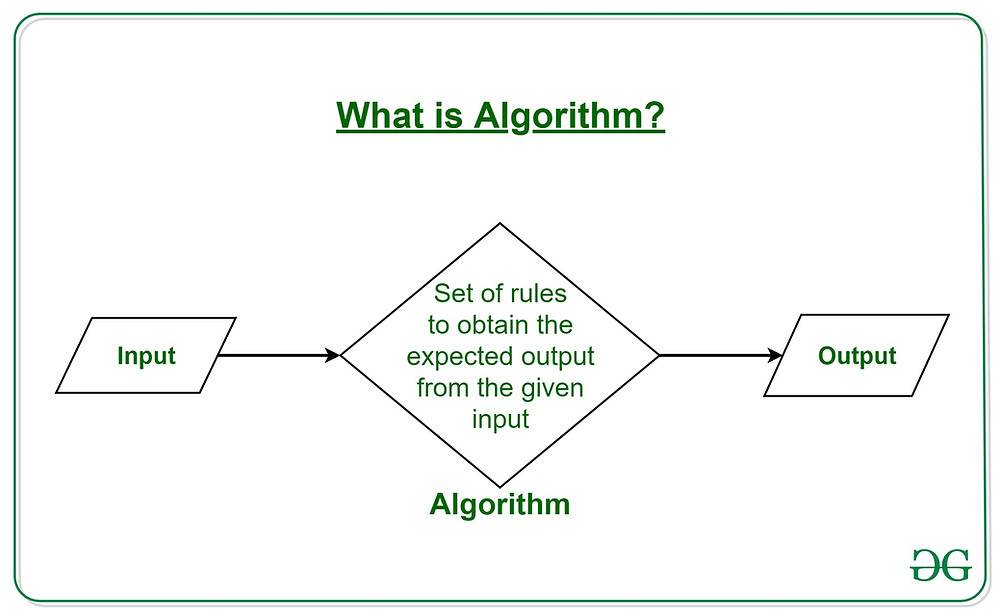Search

# List of AlgorithmsI Foundations

1 The Role of Algorithms in Computing

1.1 Algorithms

1.2 Algorithms as a technology

2 Getting Started

2.1 Insertion sort

2.2 Analyzing algorithms

2.3 Designing algorithms

3 Growth of Functions

3.1 Asymptotic notation

3.2 Standard notations and common functions

4 Divide-and-Conquer

4.1 The maximum-subarray problem

4.2 Strassen’s algorithm for matrix multiplication

4.3 The substitution method for solving recurrences

4.4 The recursion-tree method for solving recurrences

4.5 The master method for solving recurrences

4.6 Proof of the master theorem

5 Probabilistic Analysis and Randomized Algorithms

5.1 The hiring problem

5.2 Indicator random variables

5.3 Randomized algorithms

5.4 Probabilistic analysis and further uses of indicator random variables

II Sorting and Order Statistics

6 Heapsort

6.1 Heaps

6.2 Maintaining the heap property

6.3 Building a heap

6.4 The heapsort algorithm

6.5 Priority queues

7 Quicksort

7.1 Description of quicksort

7.2 Performance of quicksort

7.3 A randomized version of quicksort

7.4 Analysis of quicksort

8 Sorting in Linear Time

8.1 Lower bounds for sorting

8.2 Counting sort

8.4 Bucket sort

9 Medians and Order Statistics

9.1 Minimum and maximum

9.2 Selection in expected linear time

9.3 Selection in worst-case linear time

III Data Structures

10 Elementary Data Structures

10.1 Stacks and queues

10.3 Implementing pointers and objects

10.4 Representing rooted trees

11 Hash Tables

11.2 Hash tables

11.3 Hash functions

11.5 Perfect hashing

12 Binary Search Trees

12.1 What is a binary search tree?

12.2 Querying a binary search tree

12.3 Insertion and deletion

12.4 Randomly built binary search trees

13 Red-Black Trees

13.1 Properties of red-black trees

13.2 Rotations

13.3 Insertion

13.4 Deletion

14 Augmenting Data Structures

14.1 Dynamic order statistics

14.2 How to augment a data structure

14.3 Interval trees

IV Advanced Design and Analysis Techniques

15 Dynamic Programming

15.1 Rod cutting

15.2 Matrix-chain multiplication

15.3 Elements of dynamic programming

15.4 Longest common subsequence

15.5 Optimal binary search trees

16 Greedy Algorithms

16.1 An activity-selection problem

16.2 Elements of the greedy strategy

16.3 Huffman codes

16.4 Matroids and greedy methods

16.5 A task-scheduling problem as a matroid

17 Amortized Analysis

17.1 Aggregate analysis

17.2 The accounting method

17.3 The potential method

17.4 Dynamic tables

18 B-Trees

18.1 Definition of B-trees

18.2 Basic operations on B-trees

18.3 Deleting a key from a B-tree

19 Fibonacci Heaps

19.1 Structure of Fibonacci heaps

19.2 Mergeable-heap operations

19.3 Decreasing a key and deleting a node

19.4 Bounding the maximum degree

20 van Emde Boas Trees

20.1 Preliminary approaches

20.2 A recursive structure

20.3 The van Emde Boas tree

21 Data Structures for Disjoint Sets

21.1 Disjoint-set operations

21.2 Linked-list representation of disjoint sets

21.3 Disjoint-set forests

21.4 Analysis of union by rank with path compression

VI Graph Algorithms

22 Elementary Graph Algorithms

22.1 Representations of graphs

22.3 Depth-first search

22.4 Topological sort

22.5 Strongly connected components

23 Minimum Spanning Trees

23.1 Growing a minimum spanning tree

23.2 The algorithms of Kruskal and Prim

24 Single-Source Shortest Paths

24.1 The Bellman-Ford algorithm

24.2 Single-source shortest paths in directed acyclic graphs

24.3 Dijkstra’s algorithm

24.4 Difference constraints and shortest paths

24.5 Proofs of shortest-paths properties

25 All-Pairs Shortest Paths

25.1 Shortest paths and matrix multiplication

25.2 The Floyd-Warshall algorithm

25.3 Johnson’s algorithm for sparse graphs

26 Maximum Flow

26.1 Flow networks

26.2 The Ford-Fulkerson method

26.3 Maximum bipartite matching

26.4 Push-relabel algorithms

26.5 The relabel-to-front algorithm

VII Selected Topics

27.1 The basics of dynamic multithreading

28 Matrix Operations

28.1 Solving systems of linear equations

28.2 Inverting matrices

28.3 Symmetric positive-definite matrices and least-squares approximation

29 Linear Programming

29.1 Standard and slack forms

29.2 Formulating problems as linear programs

29.3 The simplex algorithm

29.4 Duality

29.5 The initial basic feasible solution

30 Polynomials and the FFT

30.1 Representing polynomials

30.2 The DFT and FFT

30.3 Efficient FFT implementations

31 Number-Theoretic Algorithms

31.1 Elementary number-theoretic notions

31.2 Greatest common divisor

31.3 Modular arithmetic

31.4 Solving modular linear equations

31.5 The Chinese remainder theorem

31.6 Powers of an element

31.7 The RSA public-key cryptosystem

31.8 Primality testing

31.9 Integer factorization

32 String Matching

32.1 The naive string-matching algorithm

32.2 The Rabin-Karp algorithm

32.3 String matching with finite automata

32.4 The Knuth-Morris-Pratt algorithm

33 Computational Geometry

33.1 Line-segment properties

33.2 Determining whether any pair of segments intersects

33.3 Finding the convex hull

33.4 Finding the closest pair of points

34 NP-Completeness

34.1 Polynomial time

34.2 Polynomial-time verification

34.3 NP-completeness and reducibility

34.4 NP-completeness proofs

34.5 NP-complete problems

35 Approximation Algorithms

35.1 The vertex-cover problem

35.2 The traveling-salesman problem

35.3 The set-covering problem

35.4 Randomization and linear programming

35.5 The subset-sum problem

## Recent Posts

See All

#### Drug Discovery

A drug target is a molecule in the body, usually a protein, that is intrinsically associated with a particular disease process and that could be addressed. A biological target is anything within a liv

#### DevOps

DevOps is a collaboration of the development (Dev) and operations (Ops) teams with its foundation depending on providing IT automation. DevOps is an agile methodology that includes a set of practices